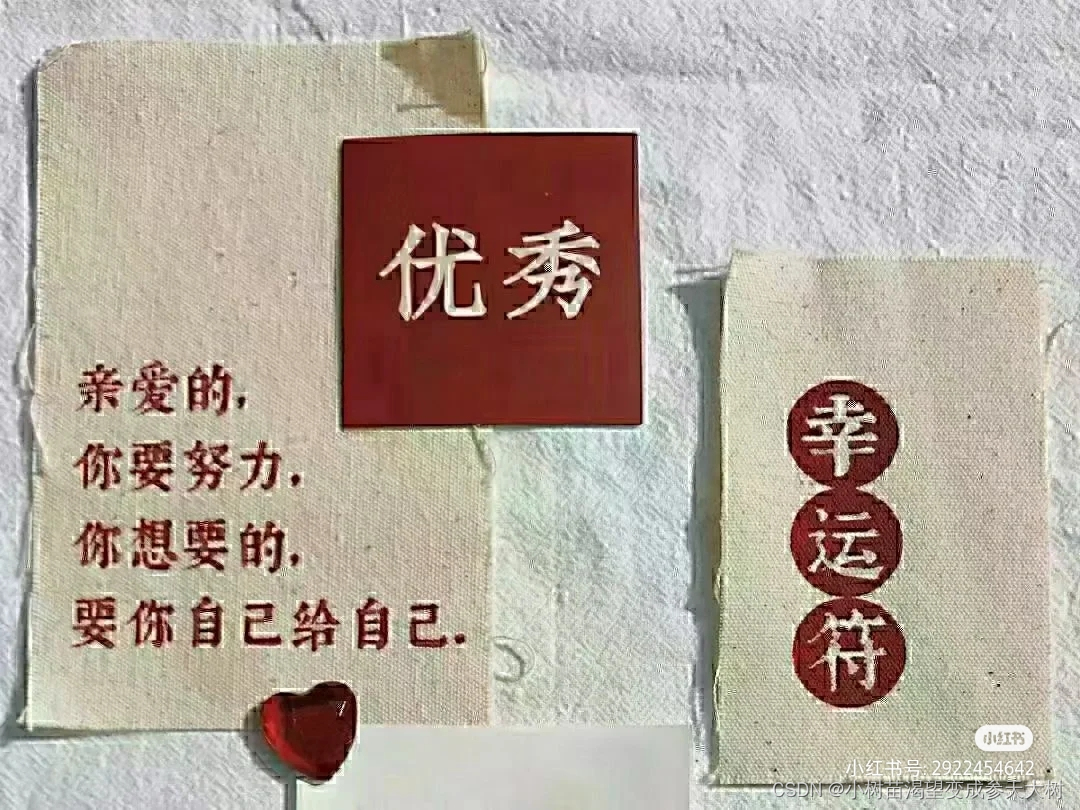# 对void的深度理解### void

• 前言
• 一、 void 关键字
• 二、 void修饰函数返回值和参数
• 三、void指针
• 3.1void * 定义的指针变量可以进行运算操作吗
• 3.2void * 用来设计通用接口
• 四、总结

# 一、 void 关键字

void通俗的来说和其他的数据类型世同级的，但是void关键字的用法非常多，他也有一些做不到的事情。

void是否可以定义变量?

``````#include <stdio.h>
#include <windows.h>
int main()
{
void a;
system("pause");
return 0;
}
``````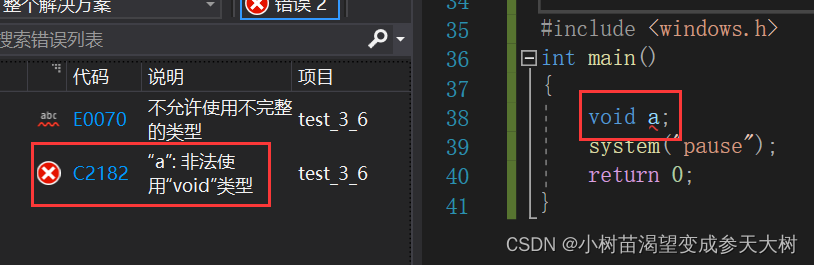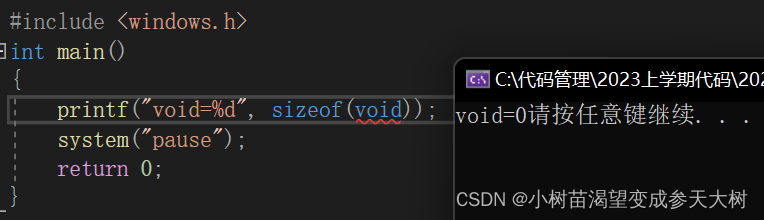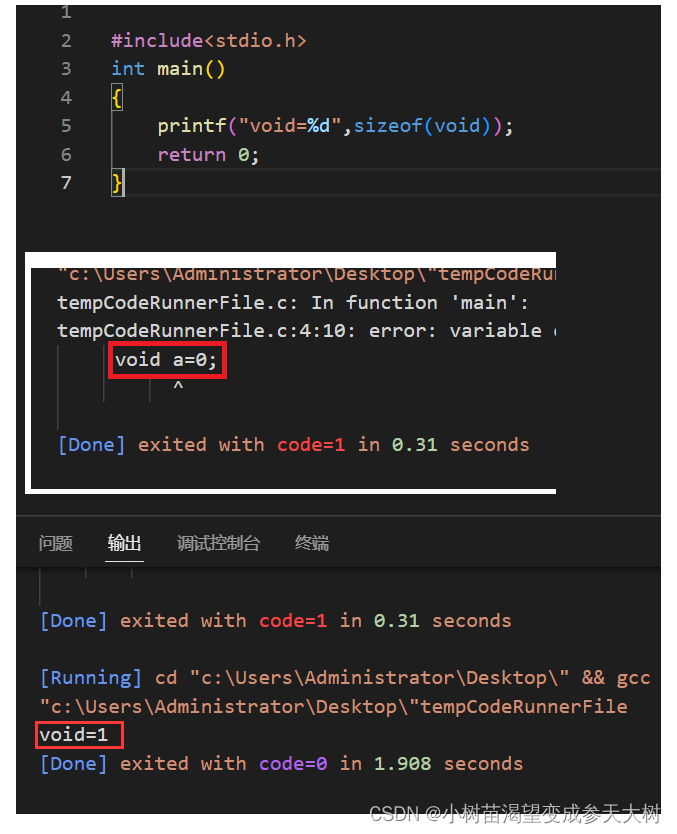void本身就被编译器视为空类型，强制的不允许定义变量

# 二、 void修饰函数返回值和参数

``````test()
{
;
}
int main()
{
int a = test();
printf("%d", a);
system("pause");
return 0;
}
``````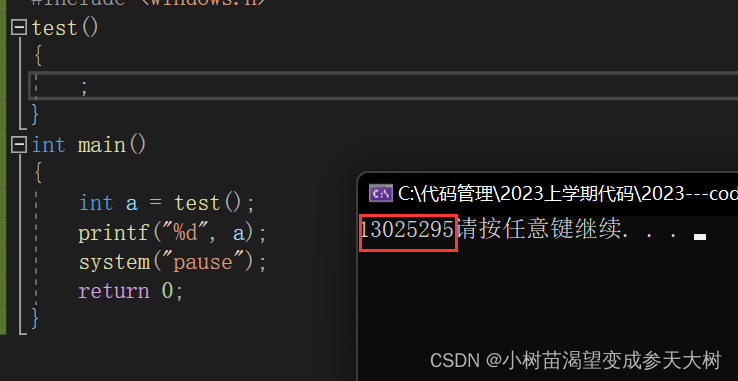``````void test()//默认不要参数
{
;
}
int main()
{
test(2,5,"niaho");
system("pause");
return 0;
}
``````

``````void test(void)//明确告诉你不要参数
{
;
}
int main()
{
test(2,5,"niaho");
system("pause");
return 0;
}
``````

1.如果一个函数没有参数，将参数列表设置成void，是一个不错的习惯，因为可以将错误明确提前发现
2.另外，阅读你代码的人，也一眼看出，不需要参数。相当于"自解释"

# 三、void指针

void不能定义变量，那么void*呢？

``````#include <stdio.h>
#include <windows.h>
int main()
{
void* p = NULL; //可以
system("pause");
return 0;
}
``````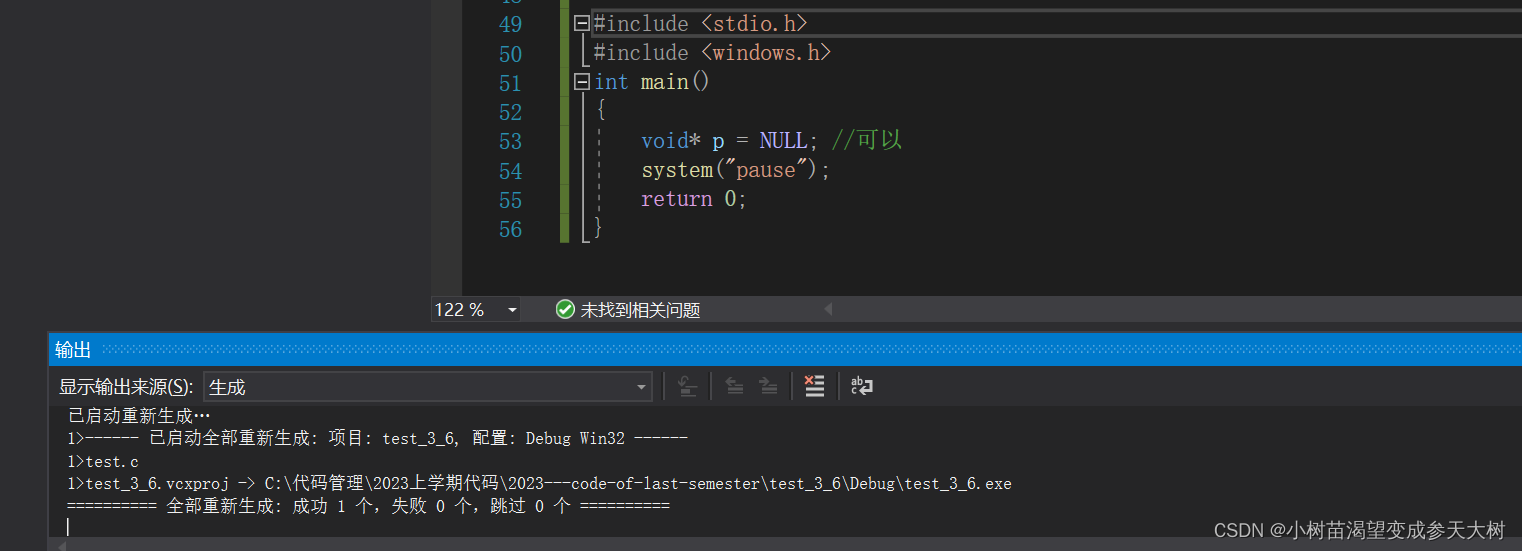``````#include <stdio.h>
#include <windows.h>
int main()
{
void *p = NULL;
int *x = NULL;
double *y = NULL;
p = x; //虽然类型不同，但是编译器并不报错
p = y; //同上
x=p;
y=p
system("pause");
return 0;
}
``````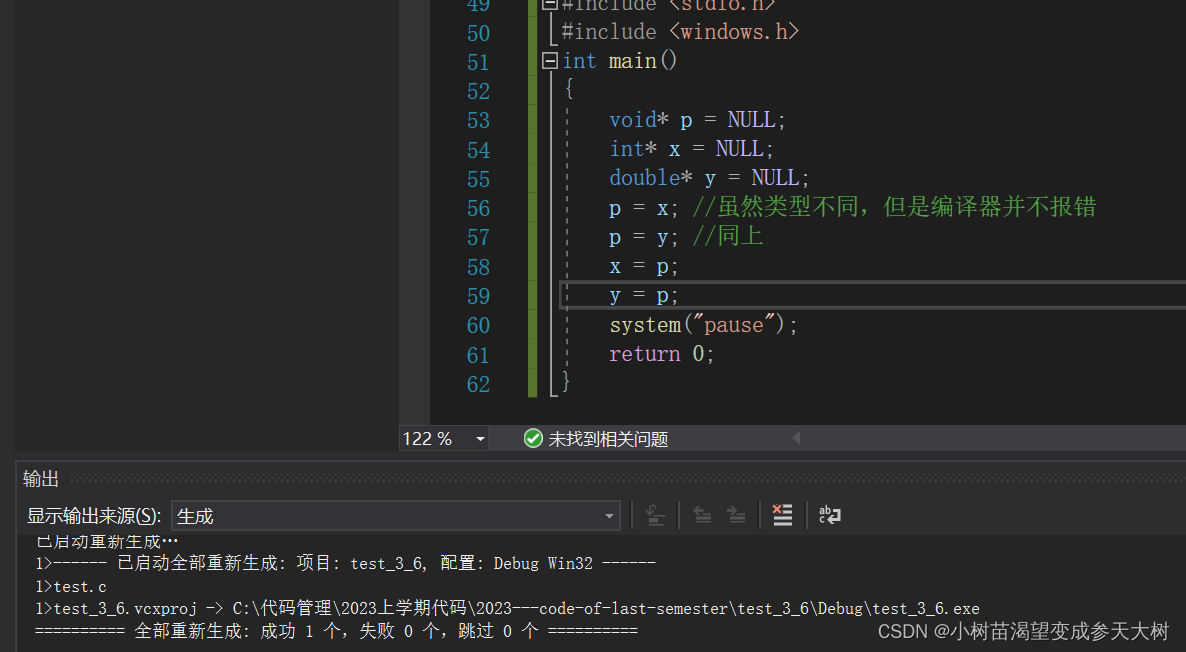1.void的作用是用来接受任意指针类型的。
2.任意指针也可以接受void

``````/void * memset ( void * ptr, int value, size_t num );
``````

## 3.1void * 定义的指针变量可以进行运算操作吗

（NULL实际就是在0进行强转变成void*类型）

``````#include <stdio.h>
#include <windows.h>
int main()
{
void *p = NULL;
p++; //报错
p += 1; //报错
system("pause");
return 0;
}
``````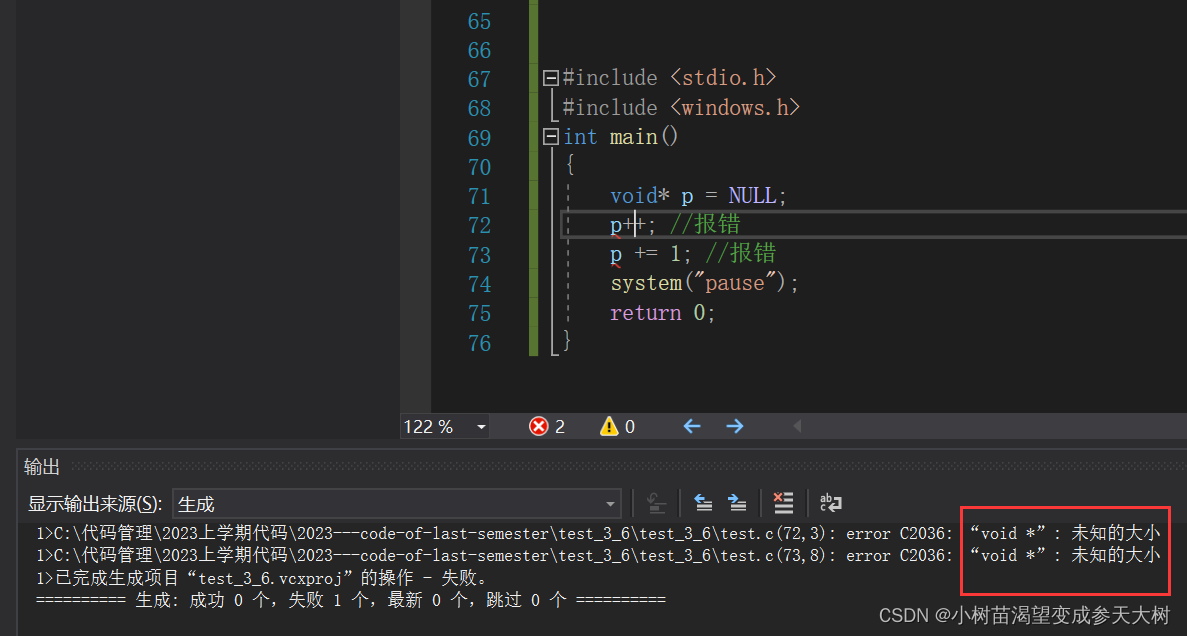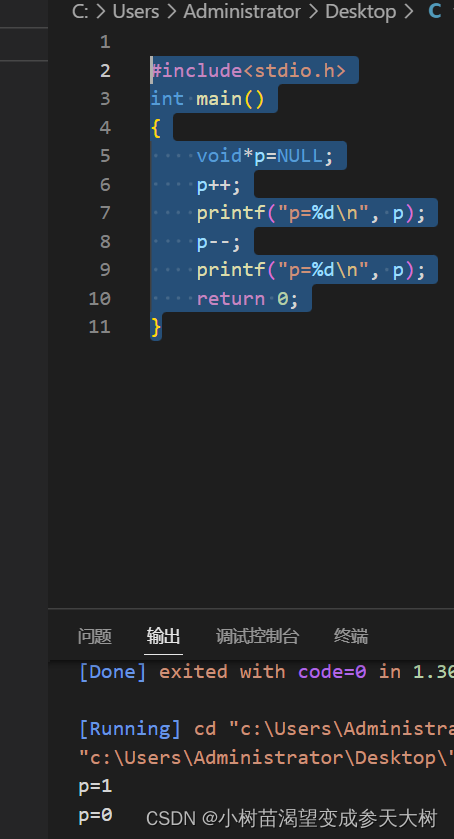qsort

# 四、总结